Deutsche VersionConversion  radians to degrees and degrees to radians ●
Type your value in a box, click your mouse anywhere on the page, or press tab key.
Power of 10 notation can be used as follows: 3.2×104 is entered 3.2e4

 Radians Degrees Parts of π RAD DEG -

The natural unit for measuring angles is the radian, which is derived from the formula for
the circumference c of a circle: c = 2 π × r, where r is the radius. If r = 1, then c = 2 π,
so the radian is defined such that a circle has 2 π radians. It also has 360 degrees.
1 radian = 360 / 2 π = 180 / π =57.295779513082320876798154814106 degrees
1 degree = 0.017453292519943295769236907684886 radians
1 arcmin = 0.000290888208665722 radians
1 arcsec = 0.00000484813681109536 radians
.

 Angle Degrees Part of Pi in numbers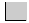90° π/2 1.570796327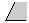60° π/3 1.047197551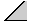45° π/4 0.785398163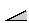30° π/6 0.523598775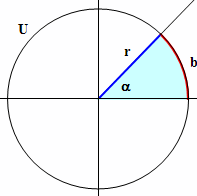Formulas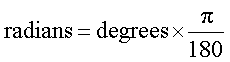Conversion from radians (RAD) to degrees (DEG):
DEG = RAD × 180 / π ~ 57.296 × RAD

Conversion from degrees (DEG) to radians (RAD):
RAD = DEG × π / 180 ~ 0.01745 × DEG

Angle Conversion

 arc seconds ("): arc minutes ('): radians (RAD): = π (pi) degrees (DEG) (°): gon, grade: percent incline, slope (%):